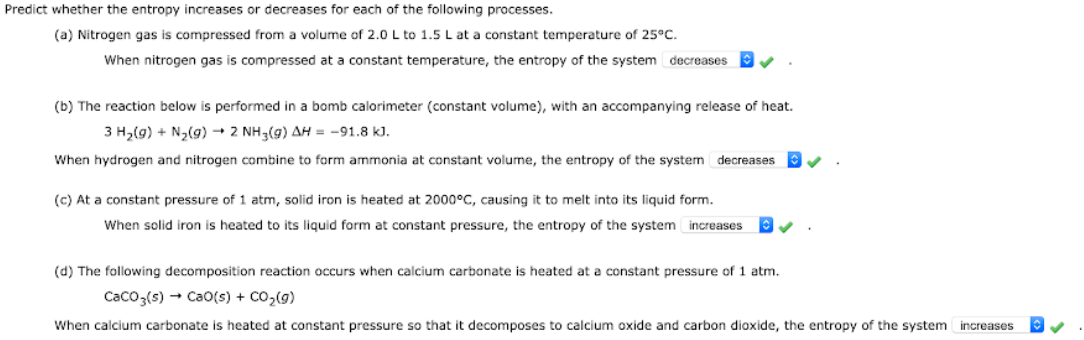# Predict whether the entropy increase or decrease for each of the following processes. (a) Nitrogen gas is compressed from a volume of 2.0 L to 1.5 L at a constant temperature of 25°C. When nitrogen gas is compressed at a constant temperature, the entropy of the system ___. (b) The reaction below is performed in a bomb calorimeter (constant volume), with an accompanying release of heat. 3 H2(g) + N2(g) -> 2 NH3(g) ΔH = -91.8 kj. When hydrogen and nitrogen combines to form ammonia at constant volume, the entropy of the system ___. (c) At constant pressure of 1 atm, solid iron is heated at 2000°C, causing it to melt into its liquid form. When solid iron is heated to its liquid form at constant pressure, the entropy of the system ___. (d) The following decomposition reaction occurs when calcium carbonate is heated at a constant pressure of 1 atm. CaCO3(s) -> CaO(s) + CO2(g) When calcium carbonate is heated at constant pressure so that it decomposes to calcium oxide and carbon dioxide, the entropy of the system ___.